Multiply Data in Excel  Step by Step Guide ▷ 2020

Basically Excel is a program that works as an array of rows and columns divided by squares and is focused on the creation, modification and management of spreadsheets. In this way, it is considered an ideal tool to effectively tabulate and organize information, as well as to insert data in order to handle, interpret and store it.

As a result, it is a valuable resource in business environments, especially in administration and accounting environments. This, due to the many functions and tools it provides to users. Thus, it is an alternative suitable for work with numerical data and perform calculations with mathematical utilities.

Therefore, Microsoft Excel allows you to do basic arithmetic calculations and use more complex mathematical functions, according to the requirements of each user. Of course, among these computations, we find the option to multiply data in cells easily. Next, we show you how.

What are the formulas for multiplying all kinds of data in Microsoft Excel?

While it is true, through the “Formulas” tab Available in the options bar of the program, you can find endless functions with which to perform very useful calculations and operations, for different purposes. In that sense, one of the most used types of formulas, are the “Mathematics and trigonometrics”. Thus, from said menu, you can get the specific function to multiply all kinds of data within a worksheet in Excel.

Which is known as “Product” or “Product” and has the ability to multiply all the numbers given as an argument, in order to return the product of the operation in question. Additionally, you can also make use of a mathematical operator set for this type of calculation. Which, is known as “Multiply” and basically it’s about use the asterisk sign . This being, a much more manual method to multiply digits inExcel

, compared to the function noted above.

Steps to multiply data in a cell in Microsoft Excel In view of what to do multiplications in Excel it’s so essential it’s worth knowinghow it should be done, correctly

.

As a consequence, we proceed to explain each of the steps to follow, in order to obtain the product of certain calculations, based on whole numbers, with decimals or with percentages:

Numbers This type of multiplication can be carried out through the function “Product” available in the menu“Formulas> Mathematics and trigonometrics”

.

• Next, we show you how to do it: To start, access the Microsoft Excel document where the numbers to be multiplied are exposed
• Or, failing that, open a blank spreadsheet and enter such data as you wish. Then, from the tab “Formulas” on the ribbon, find the Function Library group and there,click on “Mathematics and trigonometrics”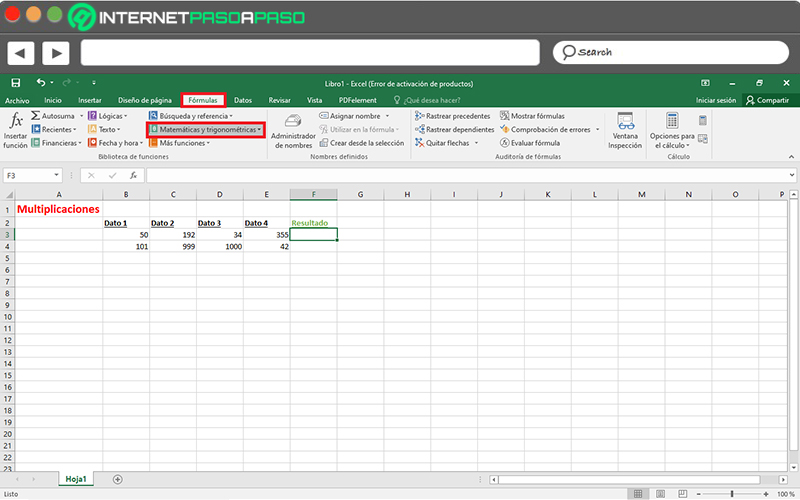• Numbers Once this is done, an extensive menu will be displayed with numerous available functions and among all of them, you have toselect the one called “PRODUCT”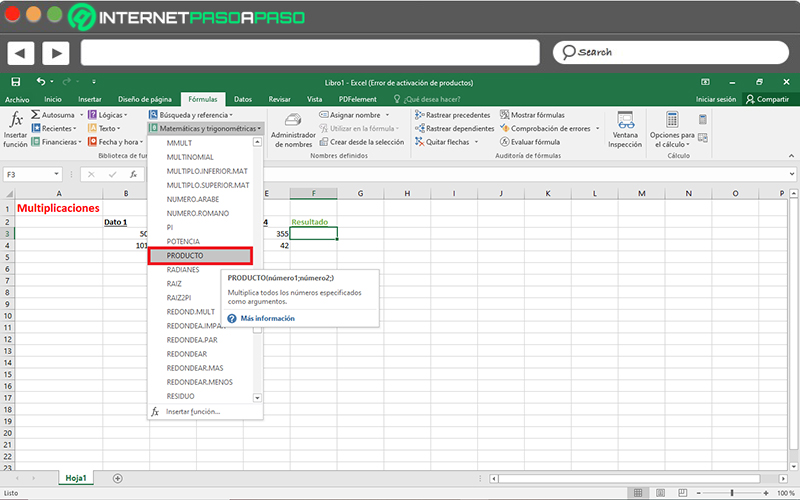• Numbers Now, a pop-up will be shown in which it will be preciseenter the arguments of the function in question . Thus the field “Number 1” is mandatory and it is thefirst number or interval you want to multiply . Meanwhile he “Number 2” is optional and consists of theAdditional numbers or ranges to multiply with the provisions of Number 1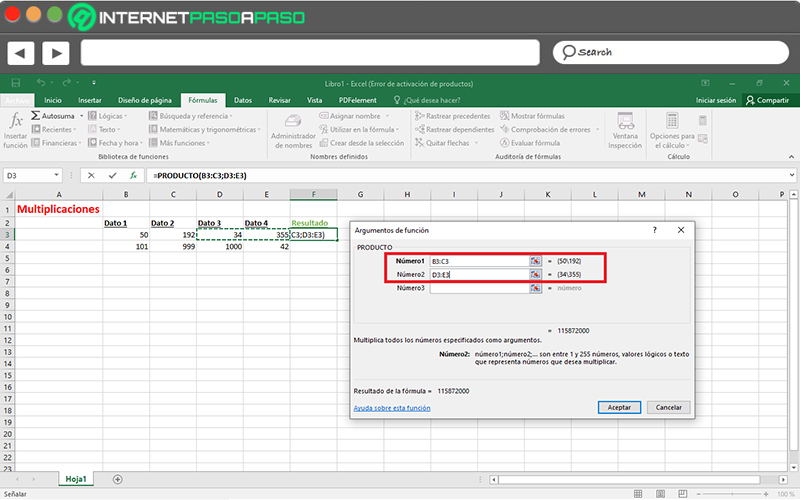• Numbers Finally, when you define the arguments of the function, you must Click on “To accept”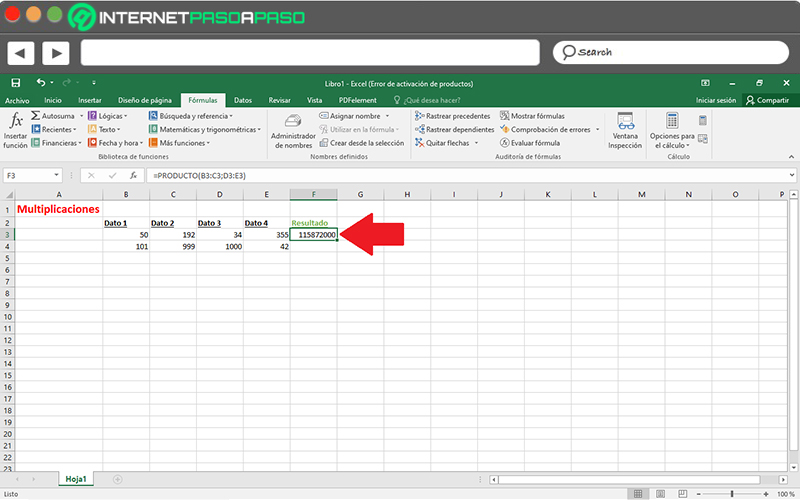Numbers It should be noted that, you can multiply up to a maximum of 255 arguments with this feature available inMicrosoft Excel

.

With Decimals When it comes to decimals, you can also perform multiplication operationsusing the “PRODUCT” function . However, there is another simpler method to obtain thistype of results in Excel

.

• Which, admits both integers as well as decimals and here we show you how to carry out this procedure: Initially, enter the Excel document that contains the decimal numerical data to multiply
• or, failing that, proceed to create a spreadsheet with those values. Thereafter, locate the cell in which you prefer to show the result of the multiplication
• and there, you need to fix the mouse cursor to start the Turkish with the mathematical operator Multiply or asterisk .Then, manually, enter the identification of each cell to be multiplied, separated by an asterisk. Specifically,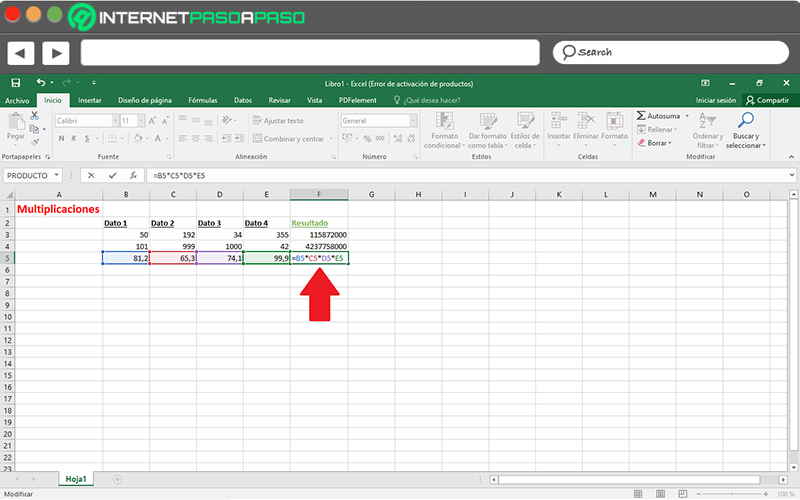• With Decimals With Decimals Once you have entered all the cells required to obtain the product,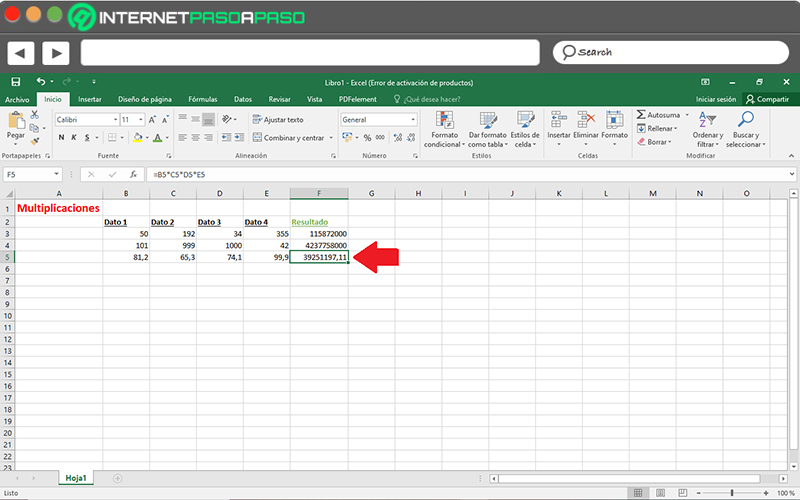With Decimals With DecimalsHowever, if the cells that you have to multiply are numerous,

it is best to use the “PRODUCT” function in Microsoft Excel

. Since, this is more practical in such cases. With Percentages In this case, the

multiplication procedure

• it is a bit different, due to the process that must be executed. However, it does not have any type of complication and so you can do it correctly. Next, we show you how to multiply data from a spreadsheet by a percentage:To exemplify this procedure, we will consider a table of numerical data that must be multiplied by a certain percentage . As a consequence, we specify these digits in a
• column and next to it, the percentage to be taken into account will be entered. Once you place all the numbers to multiply by the percentage in a column and write this percentage in an empty cell (as shown), it is necessary thatcopy said number

using the keyboard shortcut “Ctrl + C” .Having done the above, proceed to select the range of cells where the values ​​to be found are multiply. In this case,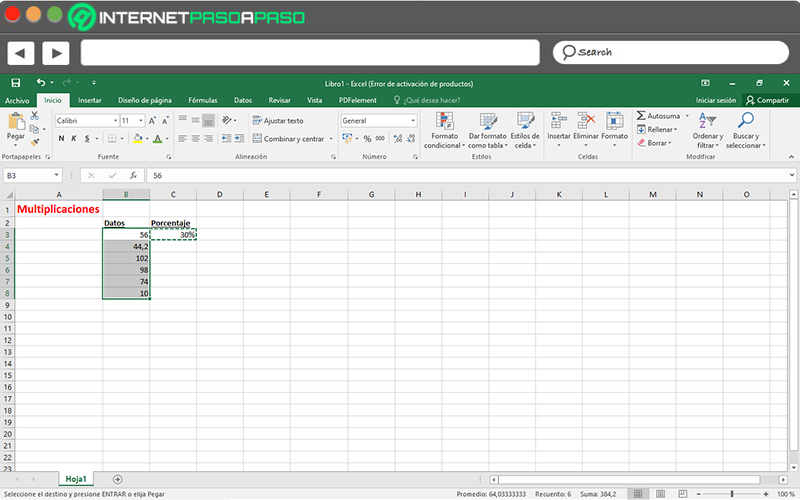• With Percentages With PercentagesNext, click the right mouse button on the cell selection and among the options displayed,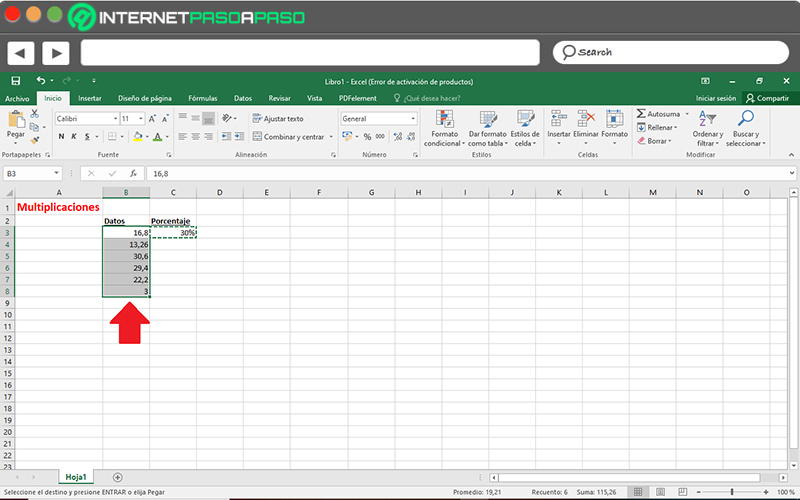With Percentages

With Percentages

If you have any questions, leave them in the comments, we will answer you as soon as possible, and it will surely be of great help to more members of the community. Thank you! 😉

Rate this post